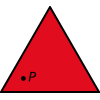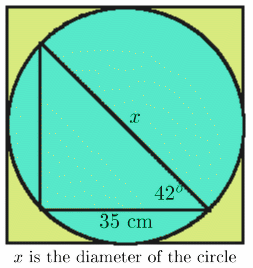#### You may also like### Fitting In

The largest square which fits into a circle is ABCD and EFGH is a square with G and H on the line CD and E and F on the circumference of the circle. Show that AB = 5EF. Similarly the largest equilateral triangle which fits into a circle is LMN and PQR is an equilateral triangle with P and Q on the line LM and R on the circumference of the circle. Show that LM = 3PQ### Triangle Midpoints

You are only given the three midpoints of the sides of a triangle. How can you construct the original triangle?### Three Way Split

Take any point P inside an equilateral triangle. Draw PA, PB and PC from P perpendicular to the sides of the triangle where A, B and C are points on the sides. Prove that PA + PB + PC is a constant.

# Farhan's Poor Square

##### Age 14 to 16 Challenge Level:Splendid solutions to Farhan's problem came in from Shabbir Telani, age 13, Jack Hunt School, Peterborough, Prav Idaikkadar,age 13, and Megan Mitchell, age 14, North London Collegiate School Maths Club, Richard Mason, Madras College, and Rachel Evans, the Mount School York. This is Shabbir's solution:

Using trigonometry:

$$x = \frac{35}{\cos 42^o} = 47.09714 = 47.10$$ (to 2 decimal places).

To find the area of the square not covered by the circle work out the area of the square minus the area of the circle.

The formula for the area is

$A = x^2 - \pi \left(\frac{x}{2}\right)^2$

So the area of the square not covered by the circle and triangle is 2218.14 - 1742.12 = 476.02 cm 2 (to 2 decimal places).